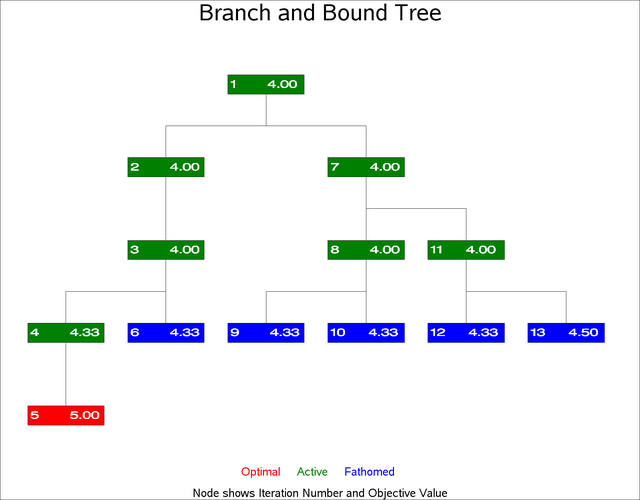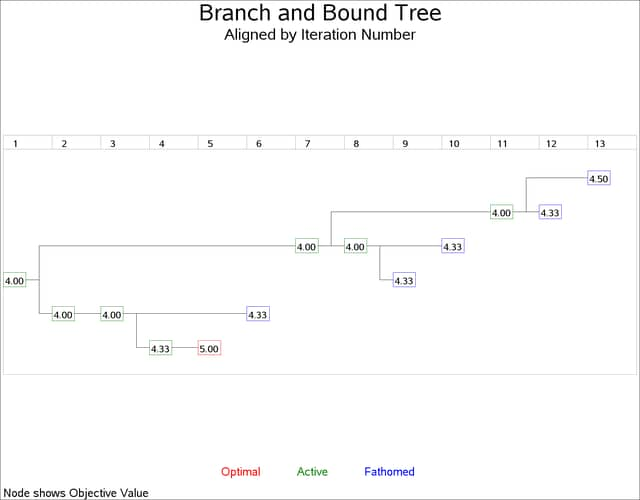Example 9.18 Branch and Bound Trees

This example illustrates a nonstandard use of PROC NETDRAW. The TREE option in PROC NETDRAW is used to draw a branch and bound tree such as one that you obtain in the solution of an integer programming problem. Refer to Chapter 6: The LP Procedure in SAS/OR 12.1 User's Guide: Mathematical Programming Legacy Procedures, for a detailed discussion of branch and bound trees. The data used in this example were obtained from one particular invocation of PROC LP.

The data set `NET` (created in the following DATA step) contains information pertaining to the branch and bound tree. Each observation of this data set represents a particular iteration of the integer program, which can be drawn as a node in the tree. The variable `node` names the problem. The variable `object` gives the objective value for that problem. The variable `problem` identifies the parent problem corresponding to each node; for example, since the second and the seventh observations have `problem` equal to '-1' and '1', respectively, it indicates that the second and the seventh problems are derived from the first iteration. Finally, the variable `_pattern` specifies the pattern of the nodes based on the status of the problem represented by the node.

```data net;
input node problem cond \$10. object;
if cond="ACTIVE"          then _pattern=1;
else if cond="SUBOPTIMAL" then _pattern=2;
else                           _pattern=3;
datalines;
1       0     ACTIVE         4
2      -1     ACTIVE         4
3       2     ACTIVE         4
4      -3     ACTIVE 4.3333333
5       4 SUBOPTIMAL         5
6       3   FATHOMED 4.3333333
7       1     ACTIVE         4
8      -7     ACTIVE         4
9      -8   FATHOMED 4.3333333
10       8   FATHOMED 4.3333333
11       7     ACTIVE         4
12     -11   FATHOMED 4.3333333
13      11   FATHOMED       4.5
;
```

The next DATA step (which creates the data set `LOGIC`) uses this child-parent information to format the precedence relationships as expected by PROC NETDRAW. Next, the two data sets are merged together to create the Network input data set (`BBTREE`) for PROC NETDRAW. The `ID` variable in the data set `BBTREE` is formatted to contain both the iteration number and the objective value.

Finally, PROC NETDRAW is invoked with the TREE, ROTATE, and ROTATETEXT options to produce a branch and bound tree shown in Output 9.18.1. Note that the ROTATE and ROTATETEXT options produce a rotated graph with a top-down orientation.

```/* set precedence relationships
using child-parent information */
data logic;
keep node succ;
set net(firstobs=2);
succ=node;
node=abs(problem);
run;
```
```proc sort data=logic;
by node;
run;
```
```/* combine the logic data and the node data */
/* set ID values                            */
data bbtree;
length id \$ 9;
merge logic net; by node;
if node < 10 then id=put(node,1.)||put(object,f8.2);
else              id=put(node,2.)||put(object,f7.2);
run;
```
```goptions border rotate=portrait;
pattern1 v=s c=green;
pattern2 v=s c=red;
pattern3 v=s c=blue;

title h=3    j=c 'Branch and Bound Tree';
title2    ' ';
footnote1 h=1.5 j=c c=red   'Optimal  '
c=green '   Active  '
c=blue  '   Fathomed ';
footnote2 ' ';
footnote3 h=1.5 ' Node shows Iteration Number and Objective Value ';
proc netdraw data=bbtree graphics out=bbout;
actnet /activity=node
successor=succ
id=(id)
nodefid
nolabel
ctext=white
coutline=black
carcs=black
xbetween=15
ybetween=3
compress
font=swiss
rectilinear
tree
rotate
rotatetext
htext=2;
run;
```

Output 9.18.1: Branch and Bound TreeIn the next invocation, PROC NETDRAW uses a modified layout of the nodes to produce a diagram where the nodes are aligned according to the iteration number. The following program uses the Layout data set produced in the previous invocation of PROC NETDRAW. The same y coordinates are used; but the x coordinates are changed to equal the iteration number. Further, the ALIGN= specification produces a time axis that labels each level of the diagram with the iteration number. Each node is labeled with the objective value. The resulting diagram is shown in Output 9.18.2.

```data netin;
set bbout;
if _seq_ = 0; drop _seq_ ;
_x_ = _from_;
id = substr(id, 3);
run;
```
```goptions rotate=landscape;
title h=3   'Branch and Bound Tree';
title2 h=2  'Aligned by Iteration Number';
footnote1 h=1.5 j=c  c=red   'Optimal     '
c=green '      Active     '
c=blue  '      Fathomed ';
footnote2 ' ';
footnote3 j=l h=1.5 ' Node shows Objective Value ';
pattern1 v=e c=green;
pattern2 v=e c=red;
pattern3 v=e c=blue;
proc netdraw data=netin graphics;
actnet /id=(id)
ctext=black
carcs=black
align = _from_
frame
pcompress
rectilinear